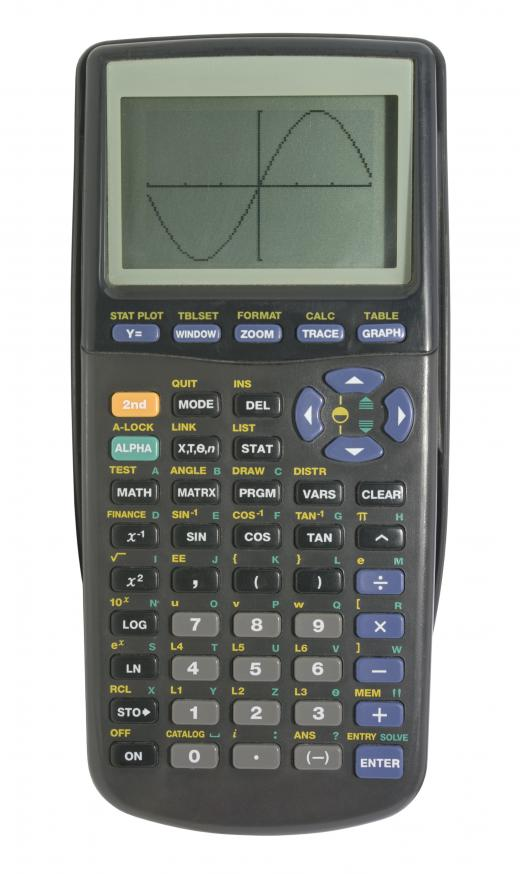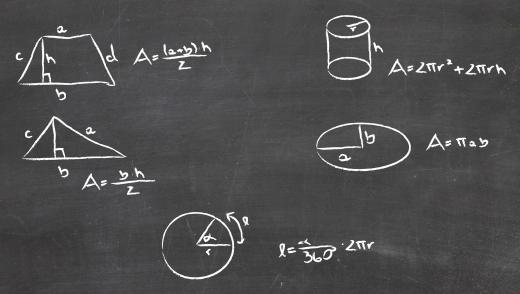# What are Irrational Numbers?Mary McMahonMary McMahon

Irrational numbers are numbers which cannot be expressed in the form of a fraction, and which are also impossible to record as a complete decimal. People have been working with irrational numbers since Greek and Roman times, and a number have been identified by mathematicians through the ages. There are a number of interesting applications and uses for irrational numbers, ranging from frustrating math students to completing complex equations.Graphing calculators may be used to work with irrational numbers.

So-called rational numbers can all be written in decimal form or the form of a fraction. ¾, for example, is a rational number, which can also be expressed as .75. When a number is irrational, it cannot be written out as a fraction with integers and the number will be impossible to record in decimal form. Pi is a famous example of an irrational number; while it is often simplified to 3.14 for the purpose of rough calculations, pi cannot actually be fully written out in decimal form because the decimal is endless.Pi is used to compute the area and circumference of circles.

Some other examples include the square root of two, Euler's number, and the golden ratio. For the purpose of simplicity, some of these numbers are written out as symbols, as in the case of “e” for Euler's number, and sometimes they will be represented in partial decimal form. When an irrational number is presented in decimal form, ellipses are usually used after the last number in the decimal to indicate that it continues, as in 3.14... for pi.

People often start working with these numbers at a young age, although they may not be introduced specifically to the concepts of rational and irrational numbers until later. Pi is one of the earliest irrational numbers which many people learn, because it is used in equations to find the area and circumference of a circle, and these equations often make an excellent introduction to more advanced mathematics for young children. People are also introduced to the concept in many of the sciences as they start to learn about equations which are commonly used.

These unusual numbers can be difficult to work with on a basic calculator, because of the limitations of the calculator. It is usually necessary to have an advanced scientific or graphing calculators which has been programmed with these numbers and their values.

Some mathematicians make the study of these numbers their life's work. These numbers often have a number of intriguing properties which are fun to explore for people who love mathematics, and a mathematician may also be able to come up with a new application for an irrational number.Mary McMahon

Ever since she began contributing to the site several years ago, Mary has embraced the exciting challenge of being a AllTheScience researcher and writer. Mary has a liberal arts degree from Goddard College and spends her free time reading, cooking, and exploring the great outdoors.Mary McMahon

Ever since she began contributing to the site several years ago, Mary has embraced the exciting challenge of being a AllTheScience researcher and writer. Mary has a liberal arts degree from Goddard College and spends her free time reading, cooking, and exploring the great outdoors.anon243373

"ellipses" is the plural form of two different words: ellipsis (dot dot dot) and ellipse (compressed circle, conic section).

amypollick

@Anon89659: I have nothing but respect for folks like you who understand this kind of math. As for me, it makes my brain hurt. But I'm glad people like you do understand it.

anon89659

There are numbers which arise in geometry and algebra and so forth which cannot be written as a fraction of whole numbers.

A good example is the length of a diagonal of a square with side length 1. This is the square root of 2 by the pythagorean theorem, and there are many proofs which show that the square root of 2 is not a fraction of whole numbers.

Another good example is the area of a circle with diameter 2. this circle has an area equal to pi, which is also not a fraction of integers, although this is much harder to prove than the first case.

the golden ratio phi, which arises in many classical geometric constructions, as well as numerous modern combinatorial problems, is also irrational. this is easy to prove: just adapt your proof that square root of 2 is irrational and note that phi=(1/2)(-1 + sqrt(5)).

the base of the natural logarithm, e, is also irrational, and this is somewhat tricky to prove. The number e is extremely important.

One interesting distinction to draw within the collection of irrational numbers is the distinction between algebraic and transcendental numbers. a number y is algebraic if you can find some polynomial p(x)=a_n(x^n)+...+a_1(x)+a_0, where each of the numbers a_n is an integer, such that p(y)=0, i.e. y is a zero of a polynomial with integer coefficients. a transcendental number is a number which is not algebraic. note that all rational numbers are trivially algebraic, as p/q is a zero of the integral polynomial q x-p.

It is fabulously difficult in general to tell whether or not a number is transcendental. liouville proved that e is transcendental, which also served as a proof that e is irrational. of the numbers listed above, the numbers sqrt{2} and phi are algebraic, while pi and e are both transcendental.

The transcendence of pi provides a proof that you cannot "square the circle" using only a compass and straightedge. this is an old geometry problem which troubled the greeks for a long time before the invention of Galois theory, a powerful and elegant algebraic theory of equations.

If you could prove that e^pi and/or pi^e are transcendental, you could probably get a job as a mathematician.

anon51828

i don't understand rational or irrational numbers. please help. i have a big test to take on this subject.